# Blender渲染App最佳实践

(1) 开通服务

(2) 地域的选择

(3) 准备OSS Bucket

## 2. 制作 Blender Docker 镜像

(1) 创建一个Dockerfile文件

``````FROM ubuntu:latest

MAINTAINER your-name<your-email>

# 更新源
RUN apt update

# 清除缓存
RUN apt autoclean

# 安装
RUN apt install python python-pip curl pulseaudio blender -y

# 启动时运行这个命令
CMD ["/bin/bash"]``````

(2) build

``docker build -t ubuntu-blender ./``

``docker images``

(3) check

``docker run -t ubuntu-blender blender -v``

`Blender 2.79 (sub 0)`

## 3. Docker镜像上传

BatchCompute支持2种Registry：阿里云的 CR（Container Registry）和阿里云的OSS。

Docker镜像上传到CR

Docker镜像上传到OSS

## 4. 创建 App

BatchCompute 提交作业，需要配置很多参数。BatchCompute 提供的 App 模板机制，让用户很方便预设参数默认值，提交作业时，只需填写少量参数即可。

(1) 开始创建 AppDocker 镜像名称，填写您已经上传到CR的镜像名称，如：`registry.cn-shenzhen.aliyuncs.com/batchcompute_test/blender:1.0`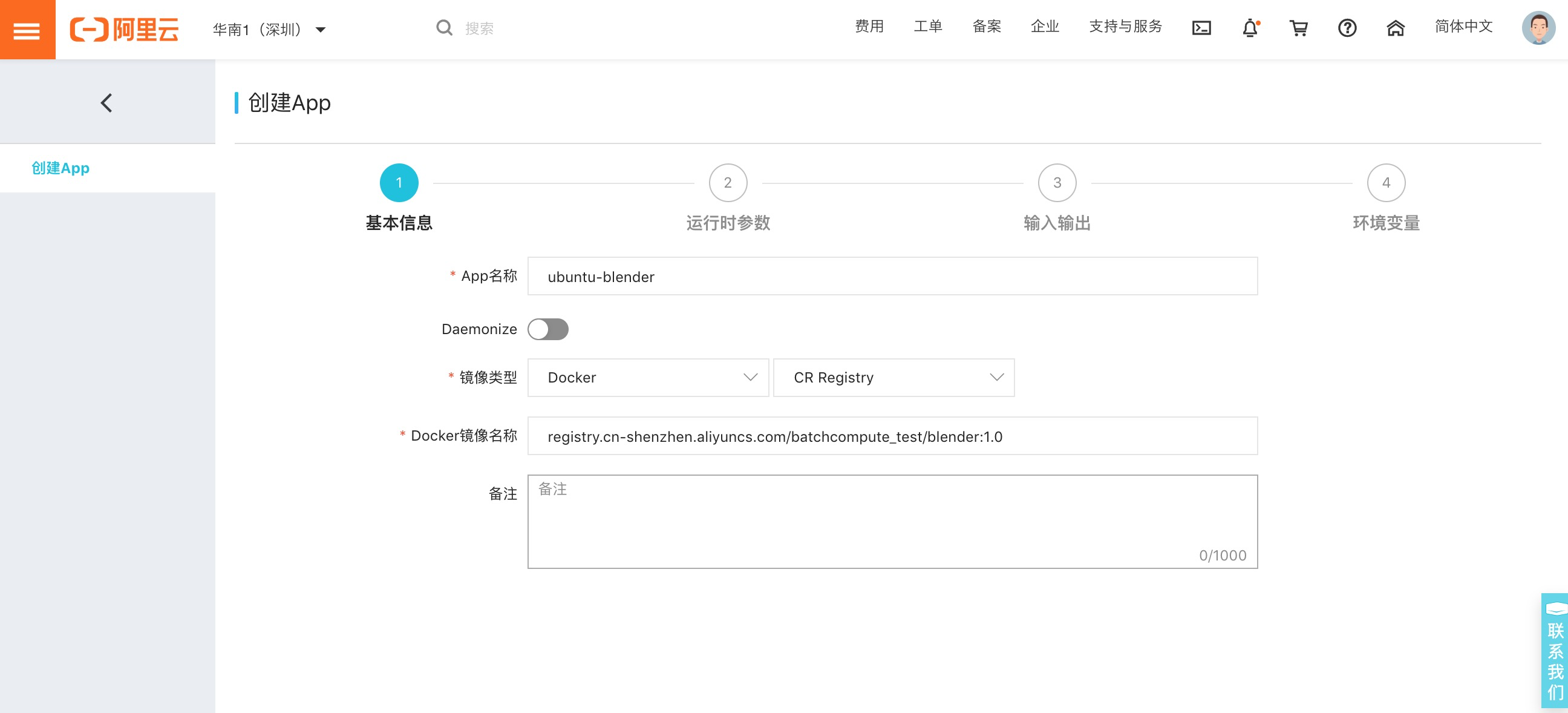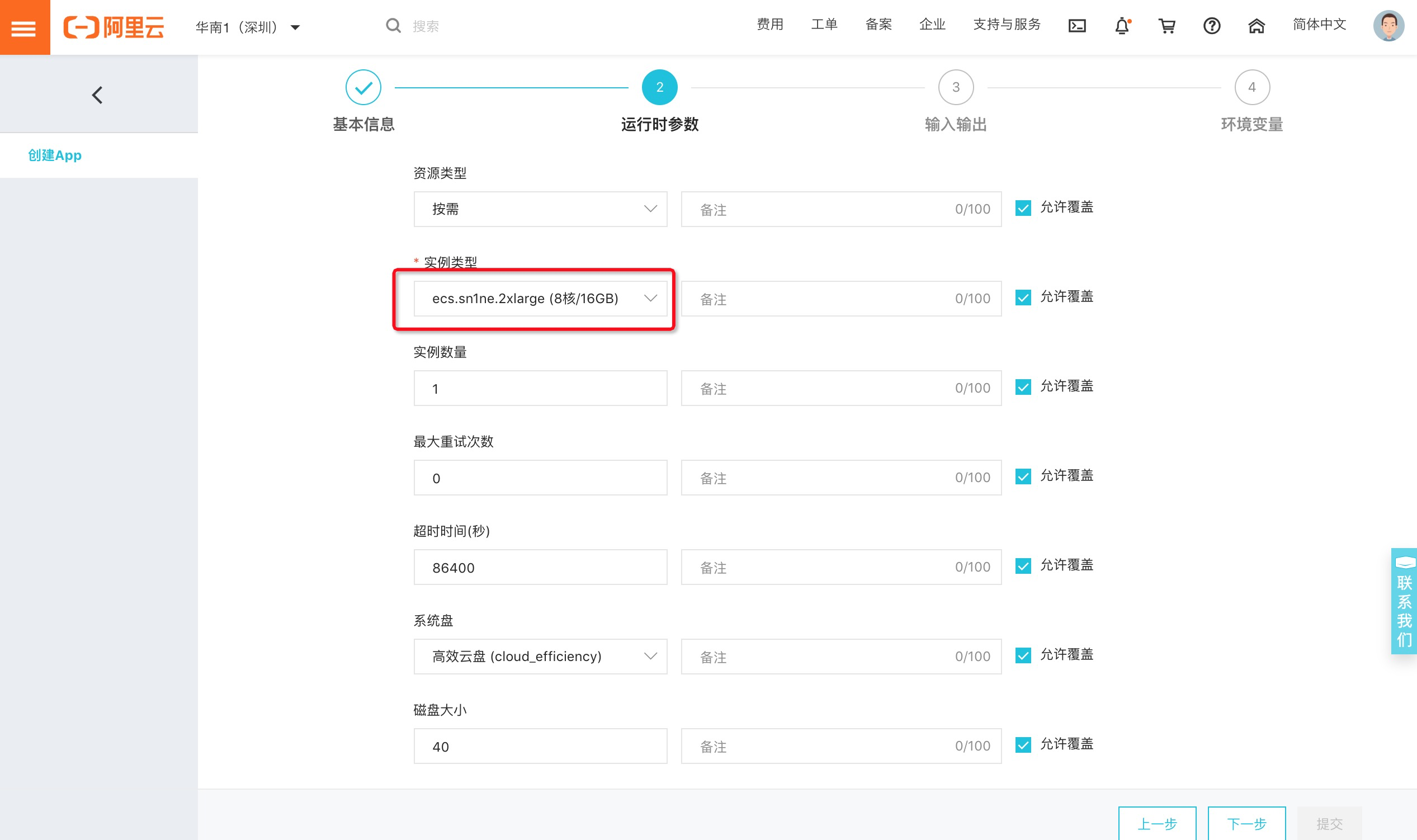(2) 命令行和参数配置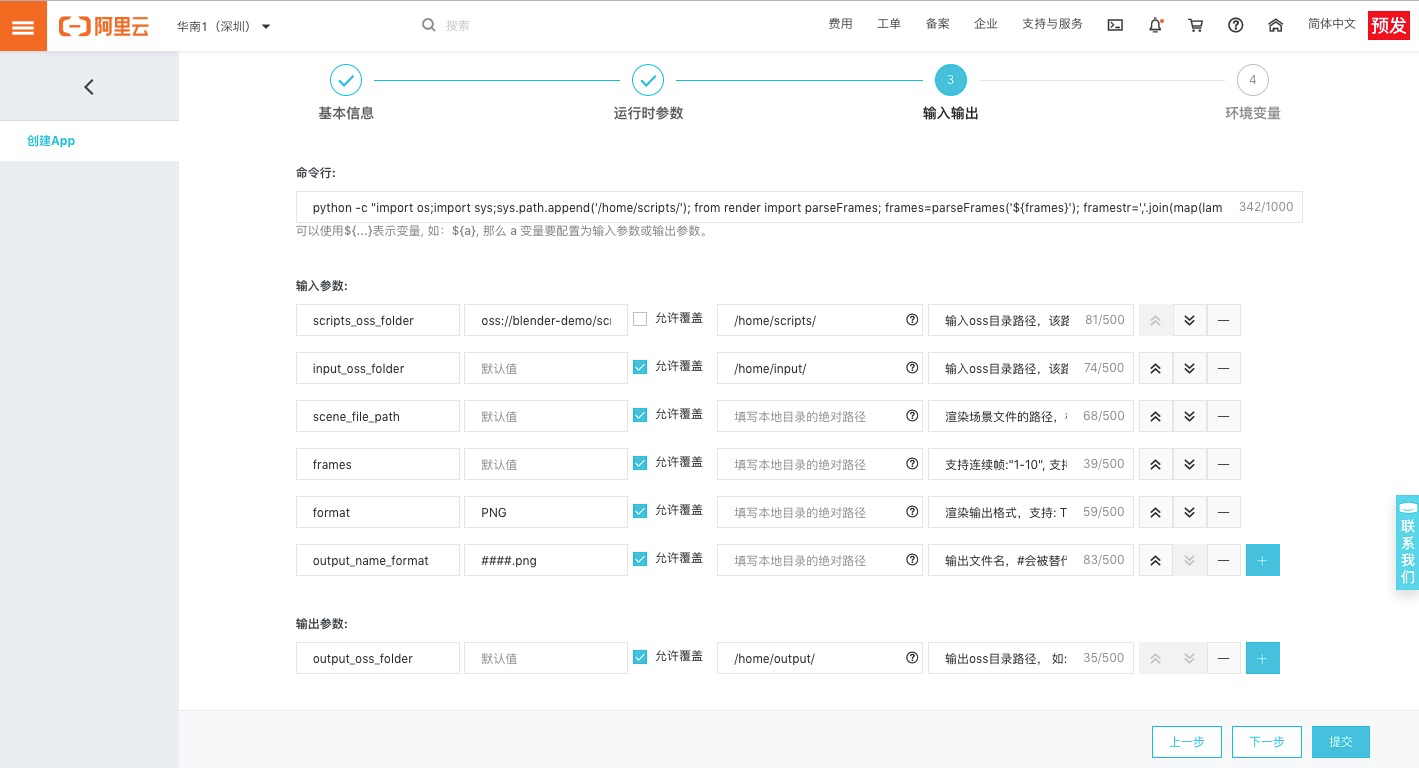``python -c "import os;import sys;sys.path.append('/home/scripts/'); from framer import parseFrames; frames=parseFrames('\${frames}'); framestr=','.join(map(lambda x:str(x), frames)); s='blender -b /home/input/\${scene_file_path} -o /home/output/result/\${output_name_format} -F \${format} -f %s' % framestr; print('exec: %s' % s); os.system(s);"``
• \${..} 都是变量，可以作为输入和输出参数。在使用此App提交作业的时候，传入的参数将替换掉这些变量。

 名称 默认值 允许覆盖 本地目录绝对路径 备注 scripts_oss_folder `oss://blender-demo/scripts/` 否 `/home/scripts/` 输入OSS目录路径，该路径将挂载到虚拟机的`/home/scripts/`，应该包含要渲染的 framer.py 文件, 如：`oss://bucket/scripts/` input_oss_folder 是 `/home/input/` 输入OSS目录路径，该路径将挂载到虚拟机的`/home/input/`，应该包含要渲染的.blend文件，如：`oss://bucket/input/` scene_file_path 是 渲染场景文件的路径，相对于`input_oss_folder`的目录路径， 如：`a.blend` 或者 `folder_name/a.blend` frames 是 支持连续帧：”1-10”, 支持多帧（逗号隔开,无空格）：”1,3,5-10” format PNG 是 渲染输出格式，支持：TGA,RAWTGA,JPEG,IRIS,IRIZ,AVIRAW,AVIJPEG,PNG,BMP output_name_format `####.png` 是 输出文件名，#会被替代为帧序号，不足位补零。举例：`test_###.png` 变成 `test_001.png`，可以在前面加目录名：`test/test_###.png`

 名称 默认值 允许覆盖 本地目录绝对路径 备注 output_oss_folder 是 `/home/output/` 输出oss目录路径， 如：`oss://bucket/output/`。

## 4. 提交渲染作业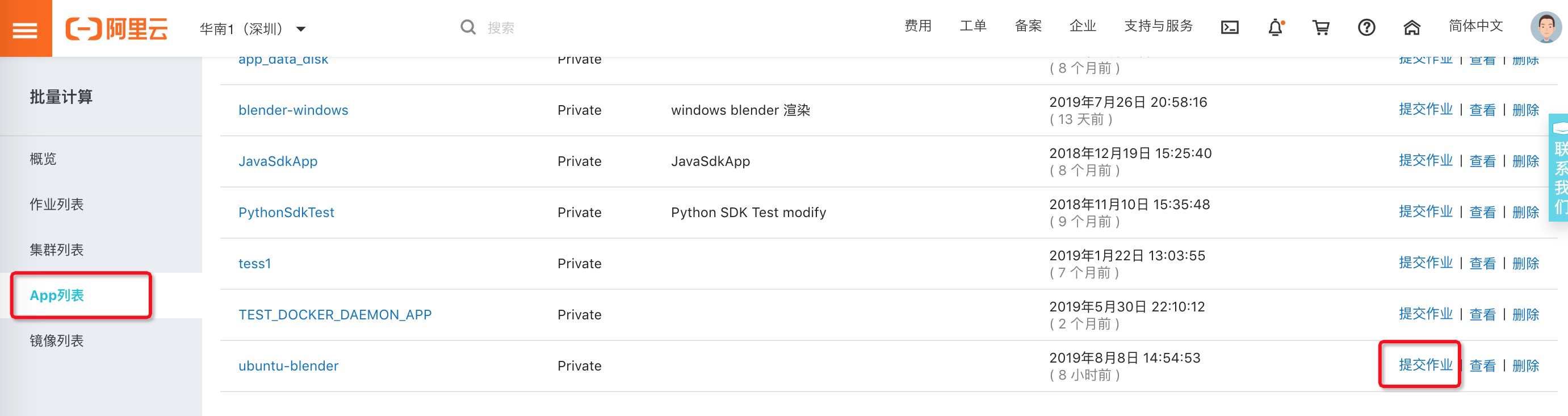(1) 准备工作(2) 开始提交作业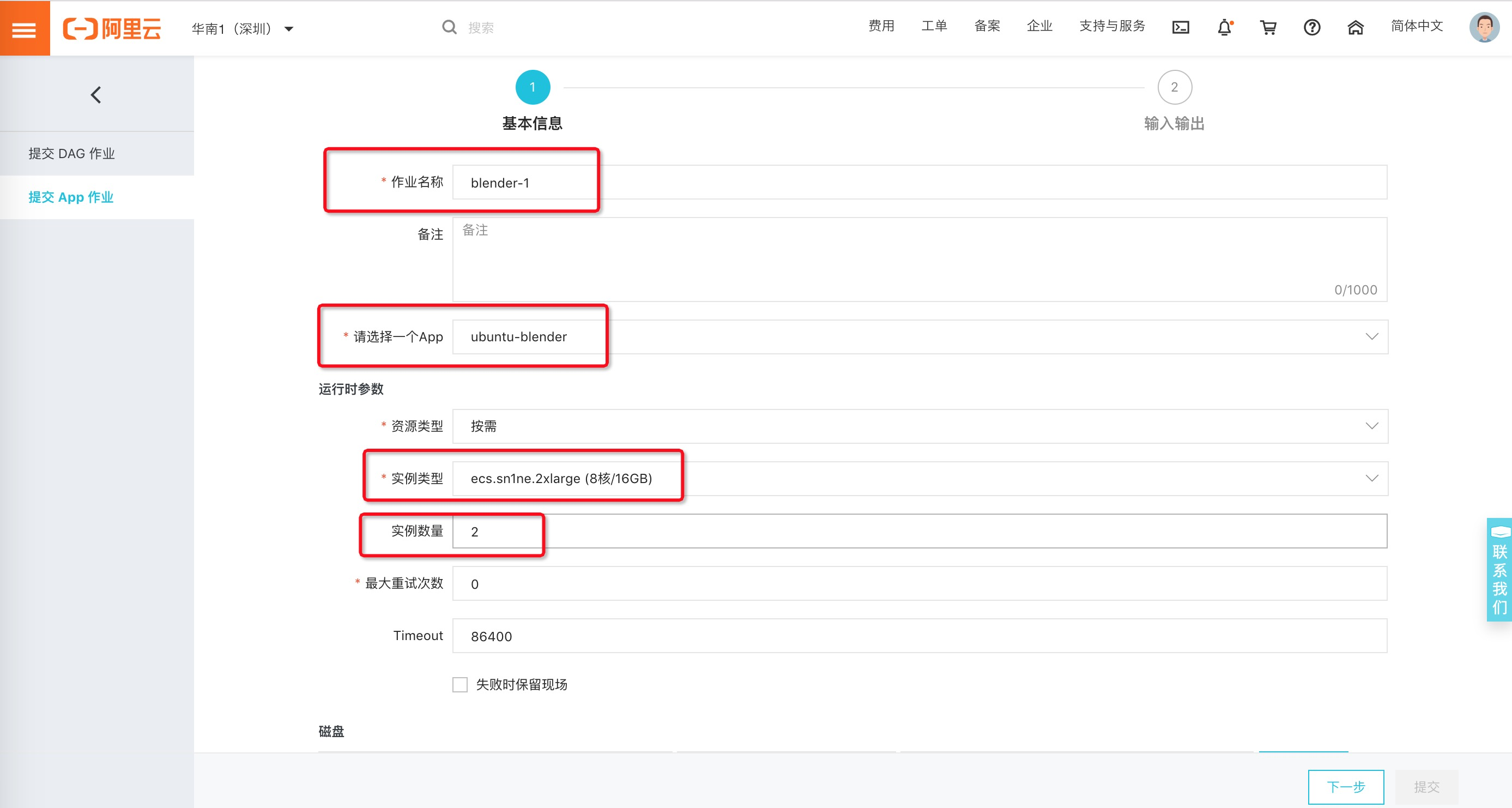• 实例类型要选大一点的，比如： 8核16GB。

• 实例数量本例子填 2 个。

(3) 参数配置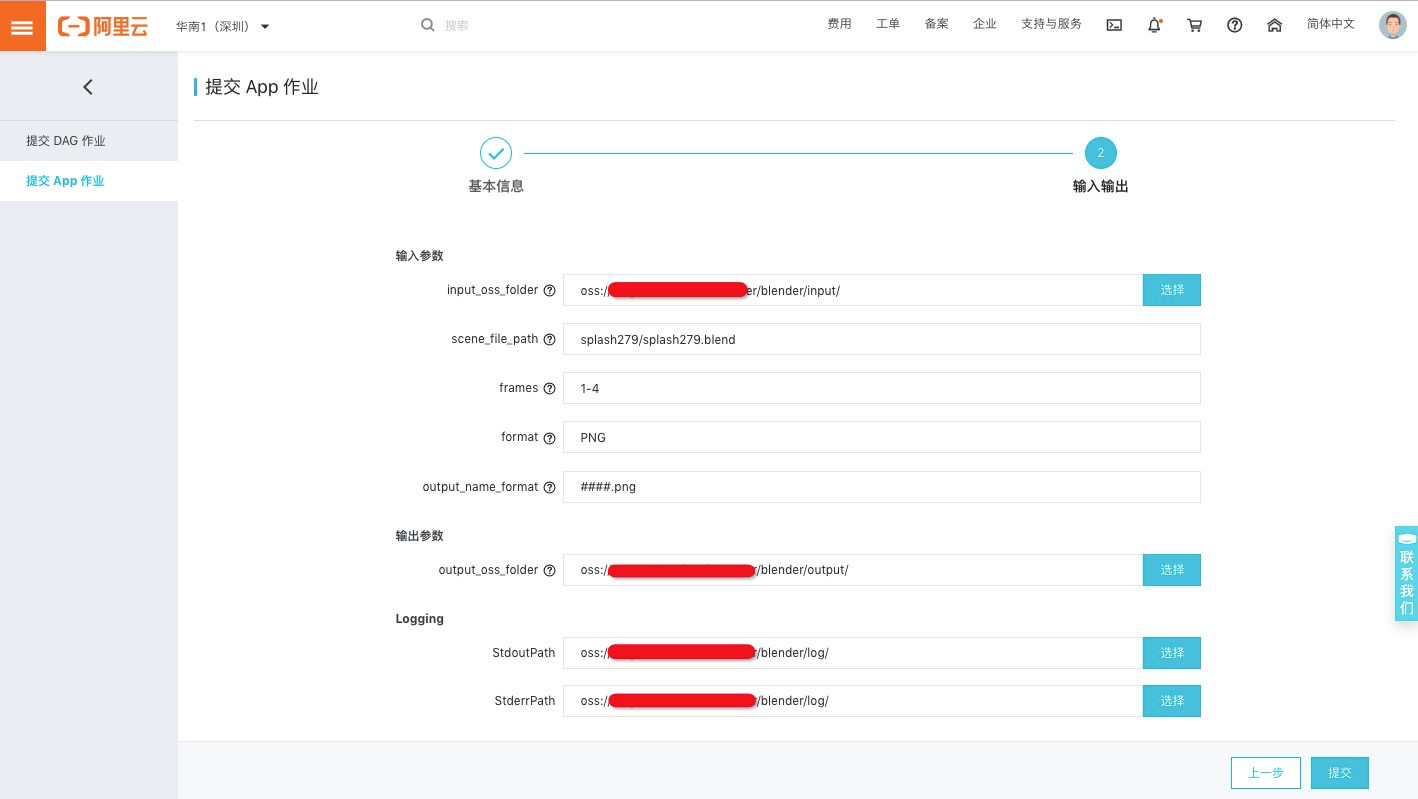参数 值 说明 input_oss_folder `oss://blender-demo/input/` 场景文件所在OSS目录 scene_file_path `splash279/splash279.blend` 场景文件名 frames `1-4` 渲染1到4帧 format PNG 渲染输出格式，默认即可 output_name_format ####.png 渲染输出文件名，默认即可
• scripts_oss_folder 设置了默认值，且不允许覆盖，可以不用填。

 参数 值 说明 input_oss_folder `oss://blender-demo/output/` 输出OSS目录, 渲染结果图片将保存到此目录的 `result/` 子目录下

Loggin（日志目录配置）：

 参数 值 说明 StdoutPath `oss://blender-demo/log/` stdout日志输出到此 StderrPath `oss://blender-demo/log/` stderr日志输出到此

## 5. 查看作业状态和结果

(1) 查看作业状态(2) 查看结果

`oss://blender-demo/output/result/`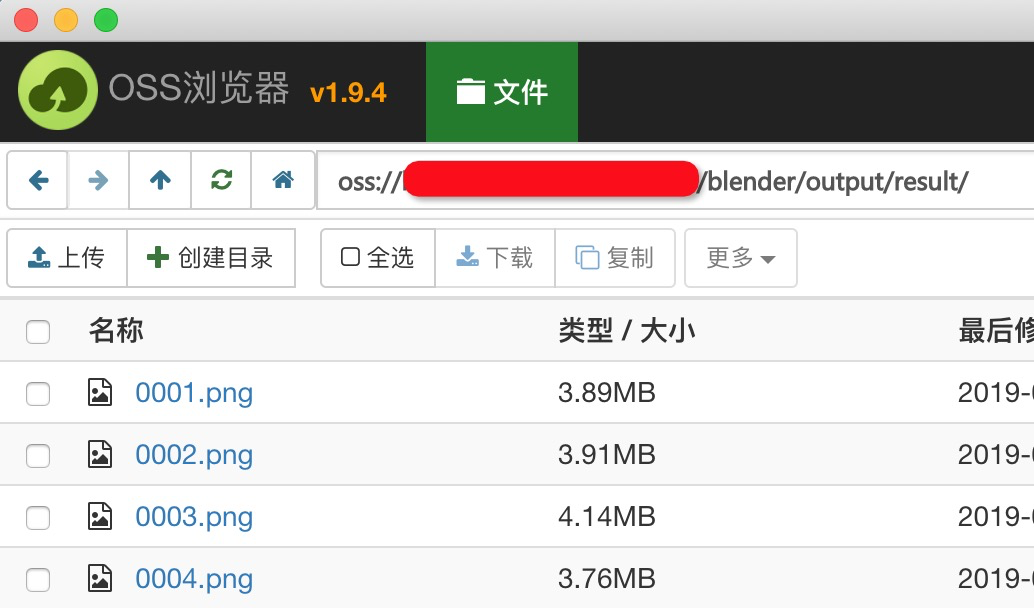(3) 渲染时长和实例规格参考

 实例规格 节点数 渲染帧数 时长 ecs.sn1ne.2xlarge(8核16GB) 2 1-4 9-12分钟 ecs.sn1ne.4xlarge (16核/32GB) 2 1-4 4-6分钟

## 6. 附录

framer.py:

``````#!/usr/bin/python
# -*- coding: UTF-8 -*-
import os
import math
import sys
import re

NOTHING_TO_DO = 'Nothing to do, exit'

def _calcRange(a,b, id, step):
start = min(id * step + a, b)
end  = min((id+1) * step + a-1, b)
return (start, end)

def _parseContinuedFrames(render_frames, total_nodes, id=None, return_type='list'):
'''
解析连续帧, 如：1-10
'''
[a,b]=render_frames.split('-')
a=int(a)
b=int(b)
#print(a,b)
step = int(math.ceil((b-a+1)*1.0/total_nodes))

#print('step:', step)
mod =  (b-a+1) % total_nodes
#print('mod:', mod)

if mod==0 or id < mod:
(start, end) = _calcRange(a,b, id, step)
#print('--->',start, end)
return (start, end) if return_type!='list' else range(start, end+1)
else:
a1 =  step * mod + a
#print('less', a1, b, id)
(start, end) = _calcRange(a1 ,b, id-mod, step-1)

#print('--->',start, end)
return (start, end)  if return_type!='list' else range(start, end+1)

def _parseIntermittentFrames(render_frames, total_nodes, id=None):
'''
解析不连续帧, 如：1,3,8-10,21
'''
a1=render_frames.split(',')
a2=[]
for n in a1:
a=n.split('-')
a2.append(range(int(a),int(a)+1) if len(a)==2 else [int(a)])

a3=[]
for n in a2:
a3=a3+n
#print('a3',a3)

step = int(math.ceil(len(a3)*1.0/total_nodes))
#print('step',step)

mod =  len(a3) % total_nodes
#print('mod:', mod)

if mod==0 or id < mod:
(start, end) = _calcRange(0, len(a3)-1, id, step)
#print(start, end)
a4= a3[start: end+1]
#print('--->', a4)
return a4
else:
#print('less',  step * mod  , len(a3)-1, id)
(start, end) = _calcRange( step * mod   ,len(a3)-1, id-mod, step-1)
if start > len(a3)-1:
print(NOTHING_TO_DO)
sys.exit(0)
#print(start, end)
a4= a3[start: end+1]
#print('--->', a4)
return a4

def parseFrames(render_frames, return_type='list', id=None, total_nodes=None):
'''
@param render_frames {string}:  需要渲染的总帧数列表范围，可以用"-"表示范围，不连续的帧可以使用","隔开, 如: 1,3,5-10
@param return_type {string}:  取值范围[list,range]。 list样例： [1,2,3], range样例： (1,3)。
注意： render_frames包含","时有效，强制为list。
@param id, 节点ID，从0开始。 正式环境不要填写，将从环境变量 BATCH_COMPUTE_DAG_INSTANCE_ID 中取得。
@param total_nodes, 总共的节点个数。正式环境不要填写，将从环境变量 BATCH_COMPUTE_DAG_INSTANCE_COUNT 中取得。
'''

if id==None:
id=os.environ['BATCH_COMPUTE_DAG_INSTANCE_ID']
if type(id)==str:
id = int(id)

if total_nodes==None:
total_nodes = os.environ['BATCH_COMPUTE_DAG_INSTANCE_COUNT']
if type(total_nodes)==str:
total_nodes = int(total_nodes)

if re.match(r'^(\d+)\-(\d+)\$',render_frames):
# 1-2
# continued frames
return _parseContinuedFrames(render_frames, total_nodes, id, return_type)

else:
# intermittent frames
return _parseIntermittentFrames(render_frames, total_nodes, id)``````